# Uncertainty Quantification with Polynomial Chaos

How to perform uncertainty quantification using polynomial chaos expansion.
Author

Murat Koptur

Published

September 13, 2022

# Introduction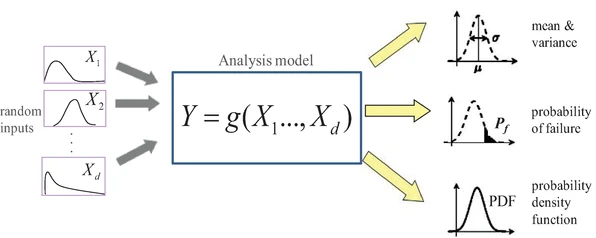Source: $$^4$$

According to $$^2$$, uncertainty quantification is defined as

The process of quantifying uncertainties associated with model calculations of true, physical QOIs, with the goals of accounting for all sources of uncertainty and quantifying the contributions of specific sources to the overall uncertainty.

How do the various sources of error and uncertainty feed into uncertainty in the model-based prediction of the quantities of interest?

# Types of uncertainties

• Aleatoric (statistical) uncertainty refers to the notion of randomness, that is, the variability in the outcome of an experiment which is due to inherently random effects $$^6$$.

• Epistemic uncertainty refers to uncertainty caused by a lack of knowledge, i.e., to the epistemic state of the agent $$^6$$.

In real life applications, both kinds of uncertainties are present.

# Types of problems

There are two major types of problems in uncertainty quantification: one is the forward propagation of uncertainty (where the various sources of uncertainty are propagated through the model to predict the overall uncertainty in the system response) and the other is the inverse assessment of model uncertainty and parameter uncertainty (where the model parameters are calibrated simultaneously using test data) $$^1$$.

Polynomial chaos is a method for quantifiying uncertainties on forward problems. Its convergence is better than Monte Carlo methods $$^3$$.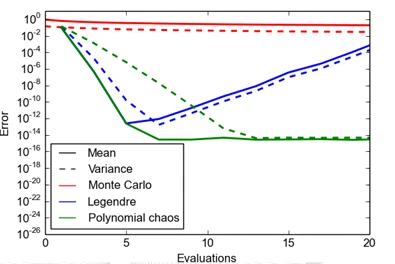Source: $$^3$$

# Polynomial Chaos Expansion (PCE)

Consider a problem in space $$x$$ and time $$t$$ where the aim is to quantify the uncertainty in response $$Y$$, computed bu a forward model $$f$$, which depends on uncertain input parameters $$Q$$:

$Y = f(x,t,Q)$

We want to quantify uncertainty in $$Y$$, but we know nothing about its density distribution $$p_Y$$. The goal is to either build the density $$p_Y$$ or revelant density properties of $$Y$$ using the density $$p_Q$$ and the forward model $$f$$ $$^5$$.

A general polynomial approximation can be defined as

$\hat{f}(x,t,Q)=\sum_{n\in I_N}c_n(x,t)\Phi_n(Q),\quad I_N=\{0,\ldots N\}$

where $$\{c_n\}_{n\in I_N}$$ are coefficients and $$\{\Phi_n\}_{n\in I_N}$$ are polynomials. If $$\hat{f}$$ is a good approximation of $$f$$, it is possible to either infer statistical properties of $$\hat{f}$$ analytically or through numerical computations where $$\hat{f}$$ is used as a surrogate for $$f$$ $$^5$$.

A polynomial chaos expansion is defined as a polynomial approximation, where the polynomials $$\{\Phi_n\}_{n\in I_N}$$ are orthogonal on a custom weighted function space $$L_Q$$:

\begin{align*} \langle \Phi_n,\Phi_m \rangle =& \mathbb{E}\Phi_n(Q)\Phi_m(Q) \\ =& \int\ldots\int \Phi_n(q)\Phi_m(q)p_Q(q)dq=0,\quad n\neq m \end{align*}

## Logistic Growth Model Example

Logistic growth model is defined as

$\frac{dX}{dt}=rX(1-\frac{X}{K})$

where $$r$$ is the growth rate and $$K$$ is the population capacity (horizontal asymptote).

Let’s define the model and visualize it for some parameters and the initial condition $$X_0=50$$:

t = numpy.linspace(0, 10, 100)
x0 = 50

def logistic_model(x, t, r, K):
return r * x * (1 - x / K)

fig, axs = plt.subplots(2,2)
fig.suptitle('Logistic model with different params')

axs = axs.ravel()

for i, params in enumerate([(0.7, 60), (1.1, 60), (0.7, 300), (1.1, 300)]):
sol = odeint(logistic_model, x0, t, args=params)
axs[i].plot(t, sol[:, 0], 'b', label='x(t)')
axs[i].legend(loc='best')
axs[i].set_xlabel('t')
axs[i].set_ylabel('x')
axs[i].grid()
axs[i].plot()

plt.show()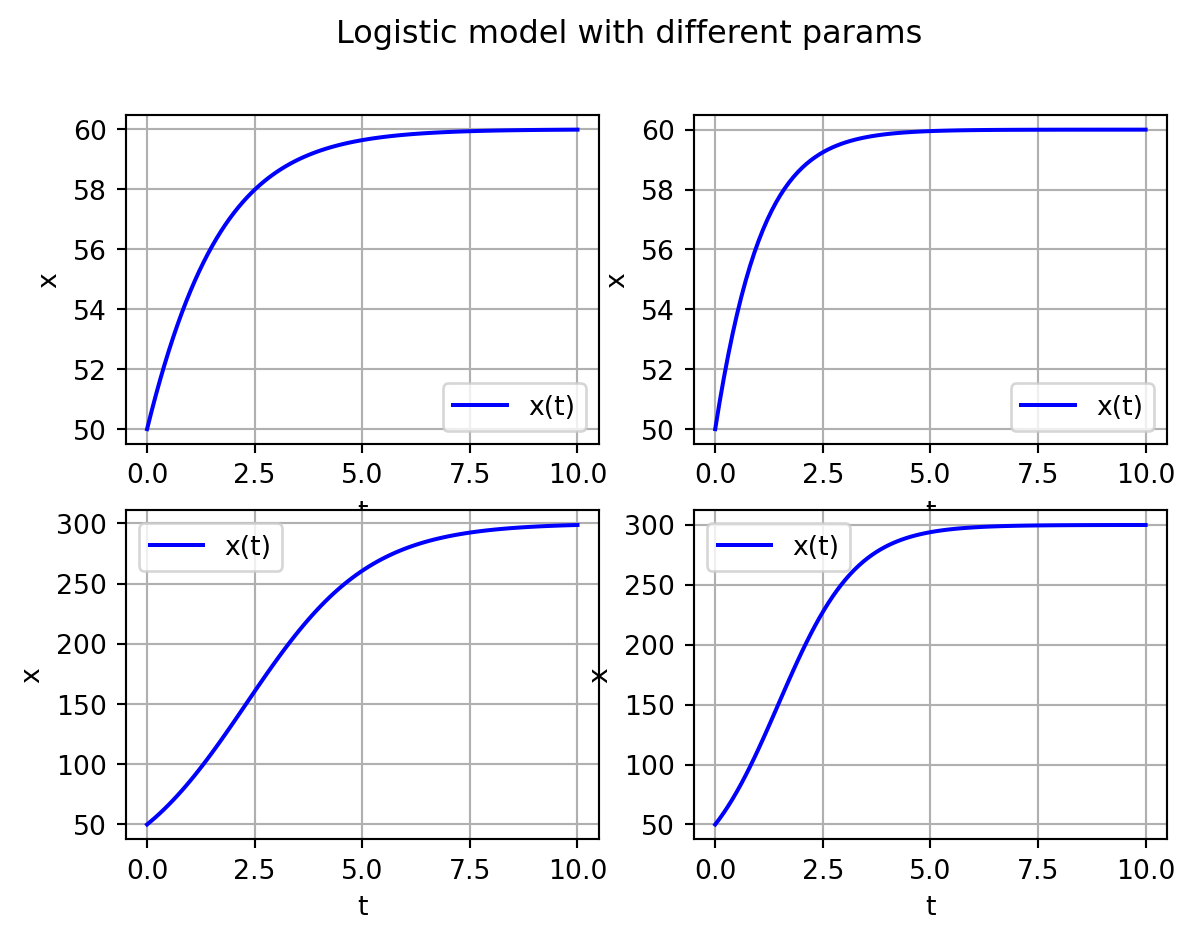Now let’s assume that we have uncertainties over our parameters and assume that

\begin{align*} r &\sim \text{Log-Normal}(1, 0.1)\\ K &\sim \text{Uniform}(100, 200) \end{align*} Let’s define our joint distribution:

rdist= chaospy.LogNormal(1, 0.1)
Kdist = chaospy.Uniform(100, 200)
joint = chaospy.J(rdist, Kdist)

grid = numpy.mgrid[joint.lower:joint.upper+1, joint.lower:joint.upper+1]
contour = plt.contourf(grid, grid, joint.pdf(grid), 50)
plt.scatter(*joint.sample(50, seed=1234))
plt.xlim(joint.lower, joint.upper)
plt.ylim(joint.lower, joint.upper)
plt.show()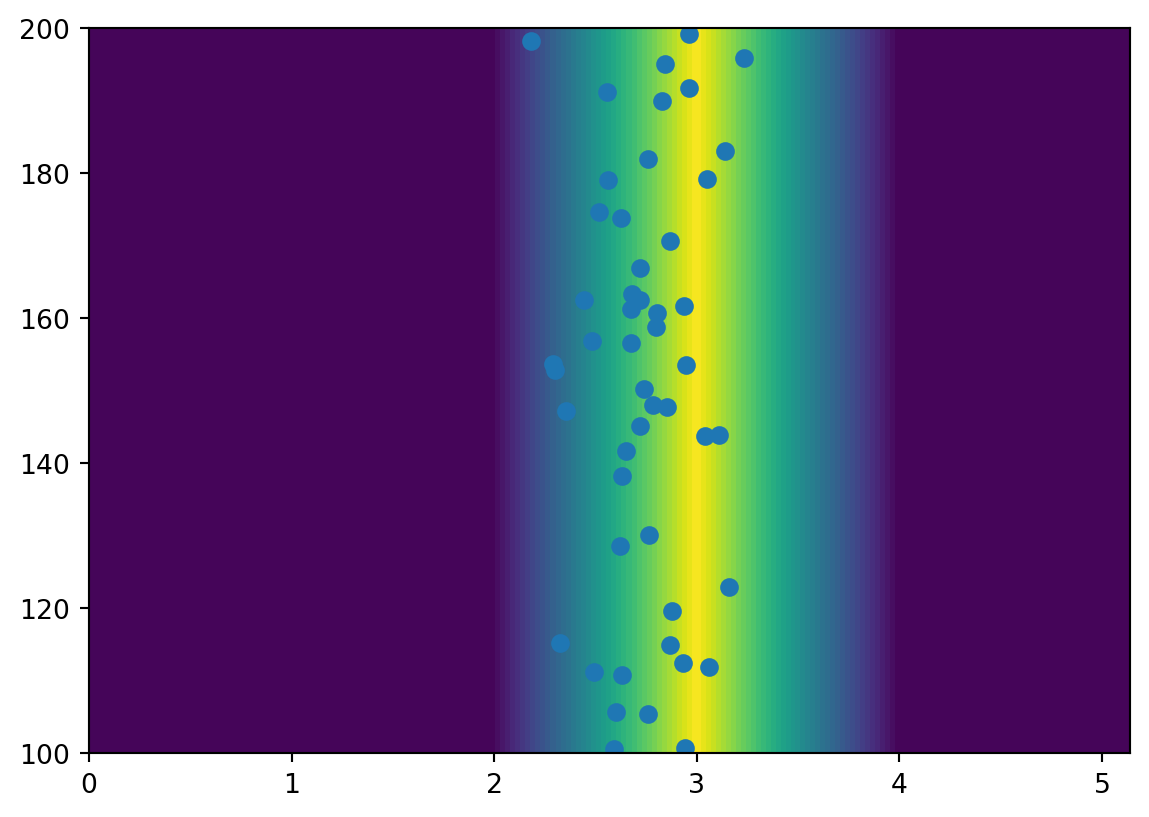Generate expension, sample the joint distribution, evaluate model at these points and plot:

expansion = chaospy.generate_expansion(order=3, dist=joint)

# and sample the joint distribution
samples = joint.sample(1000, rule="sobol")

# and evulate solver at these samples
evaluations = numpy.array([odeint(logistic_model, x0, t, args=(sample, sample)) for sample in samples.T])

# and plot
plt.plot(t, evaluations[:,:,0].T, alpha=0.1)
plt.show()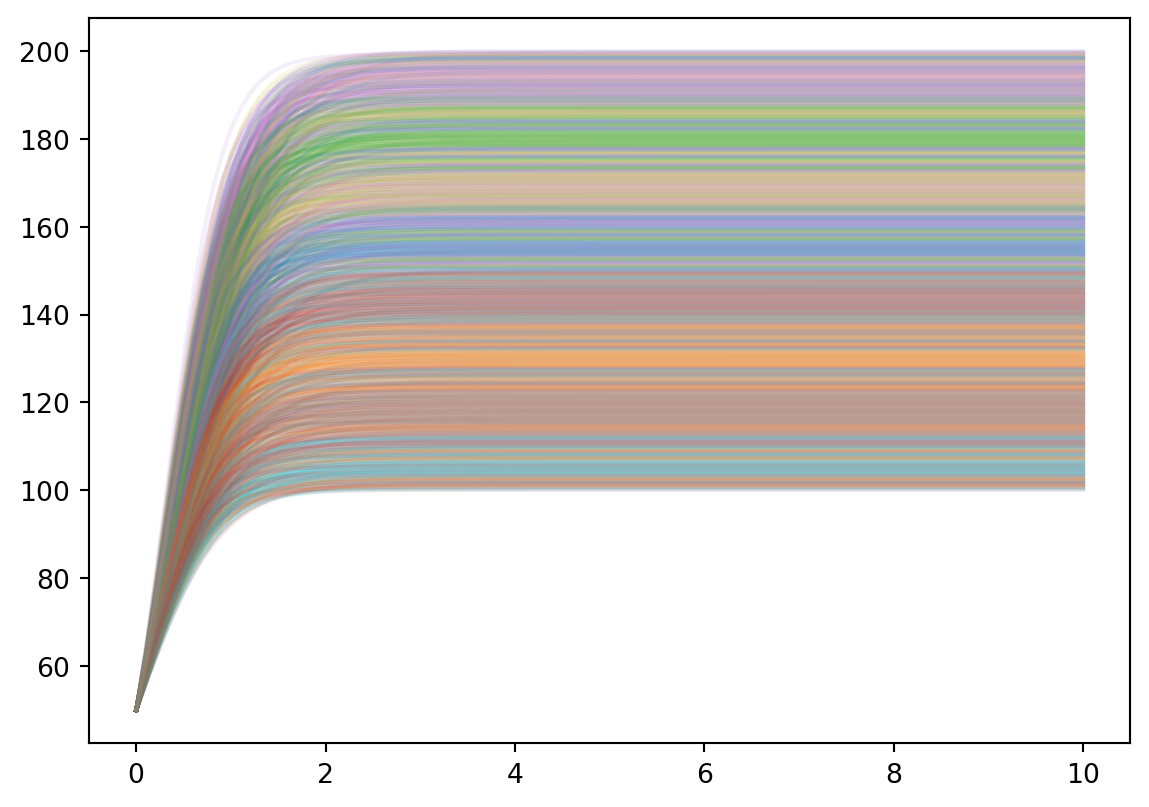Create polynomial approximation:

approx_solver = chaospy.fit_regression(expansion, samples, evaluations)

Calculate mean and deviance and plot:

expected = chaospy.E(approx_solver, joint)
deviation = chaospy.Std(approx_solver, joint)

plt.fill_between(t, expected[:,0]-2*deviation[:,0], expected[:,0]+2*deviation[:,0], alpha=0.4)
plt.plot(t, expected[:,0])
plt.show()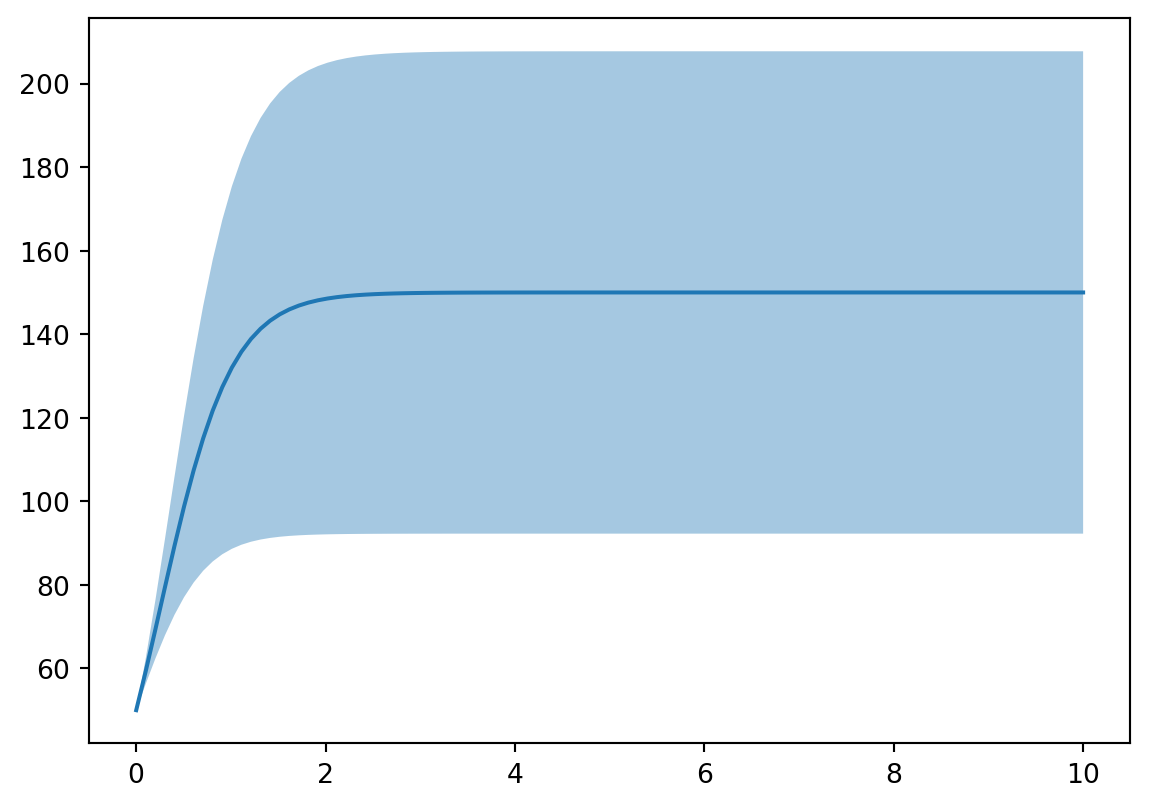## Citation

BibTeX citation:
@online{koptur2022,
author = {Murat Koptur},
title = {Uncertainty {Quantification} with {Polynomial} {Chaos}},
date = {2022-09-13},
url = {https://www.muratkoptur.com/MyDsProjects/Analysis.html},
langid = {en}
}